# 1 有监督和半监督的概念

• （直推学习）Transductive learning：无标签数据就是测试数据，没有用label，只用了feature。

• （归纳学习）Inductive Learning不把测试集考虑进来，也就是训练数据中使用的unlabelled的数据并非测试集中的数据。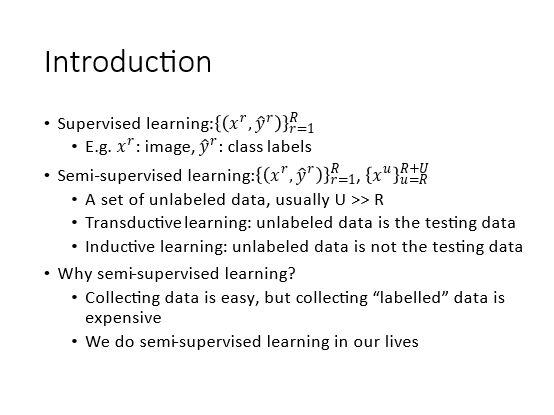如下图，假设我们要做分类的项目，要建立一个猫和狗的分类决策面，同时有一大堆有关猫和狗的图片，蓝色和橙色是有标签的，但是大部分灰色是没有标签的。那么如果只考虑有标签数据，那么决策面会是这样：但是这些灰色点没有标签，但是它们还是可以告诉我们一些信息，那么可能决策面就会变成：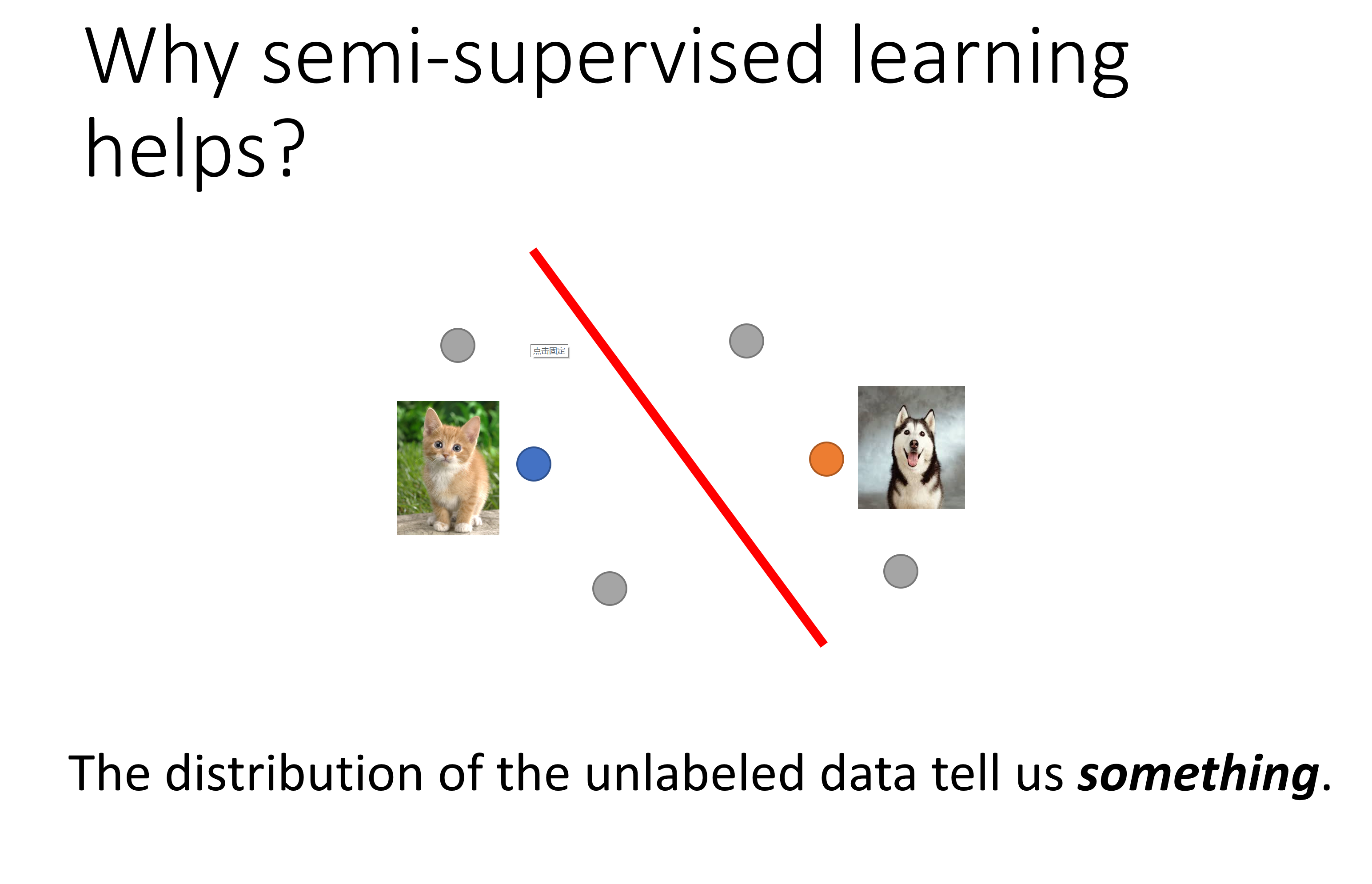但是可能实际的unlabelled data是均匀分布两边的，那么就很难分，一般跟unlabelled data的假设有关系。比如下图红框实际上是狗狗，只是绿色背景让它分布在这边：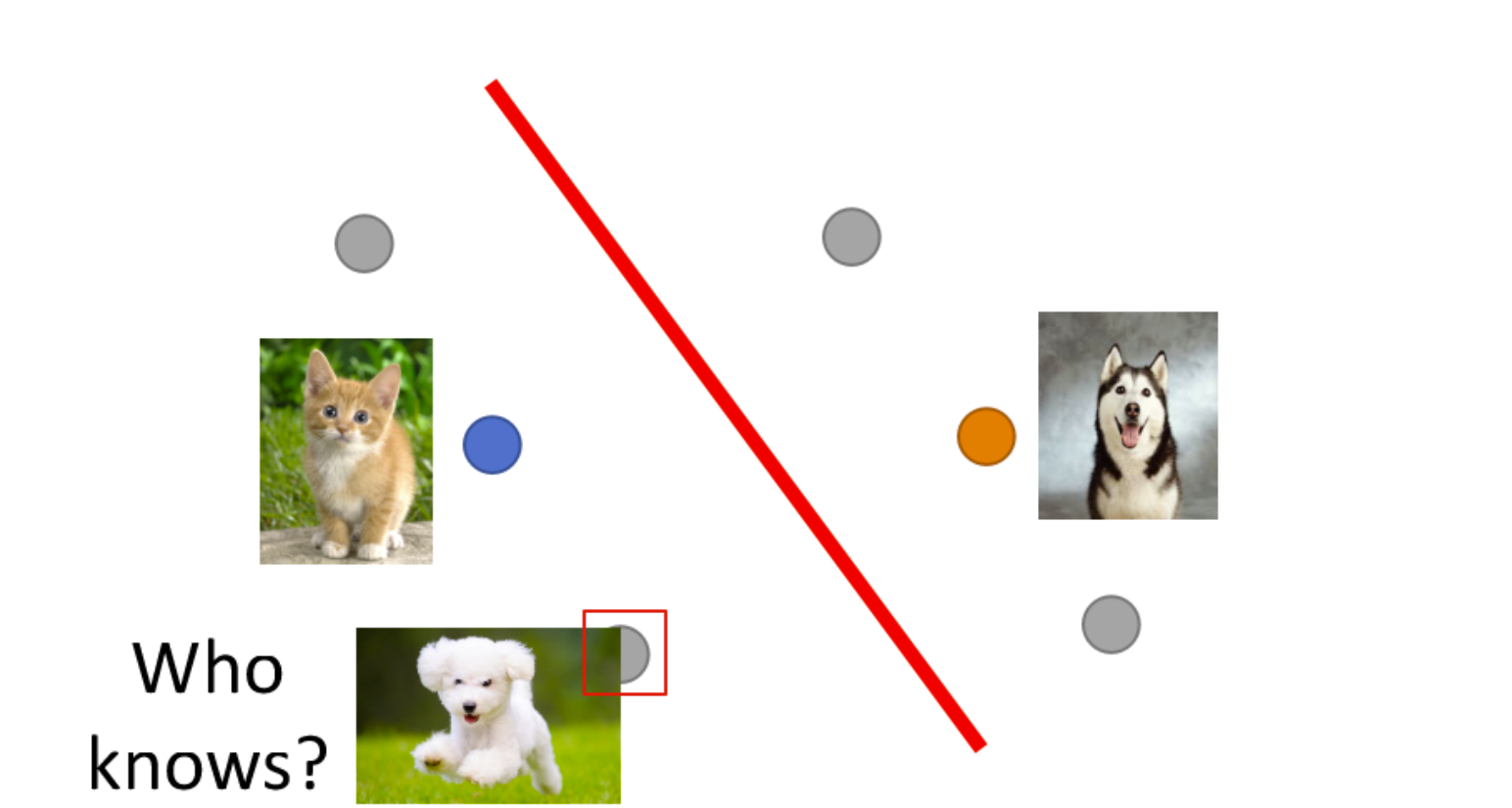# 2 半监督学习中的生成模型

## 2.1 有监督的生成模型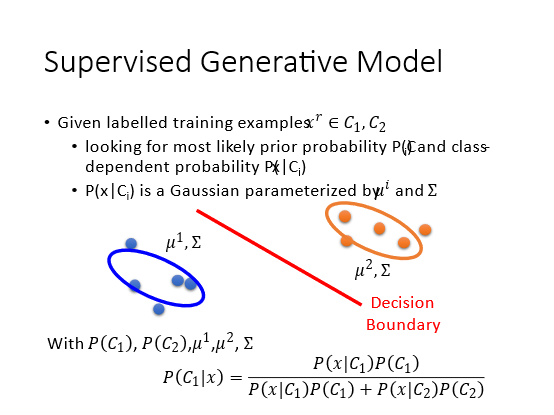P ( C 1 ∣ x ) = P ( x ∣ C 1 ) P ( C 1 ) P ( x ∣ C 1 ) P ( C 1 ) + P ( x ∣ C 2 ) P ( C 2 ) P\left(C_{1} \mid x\right)=\frac{P\left(x \mid C_{1}\right) P\left(C_{1}\right)}{P\left(x \mid C_{1}\right) P\left(C_{1}\right)+P\left(x \mid C_{2}\right) P\left(C_{2}\right)}

## 2.2 半监督的生成模型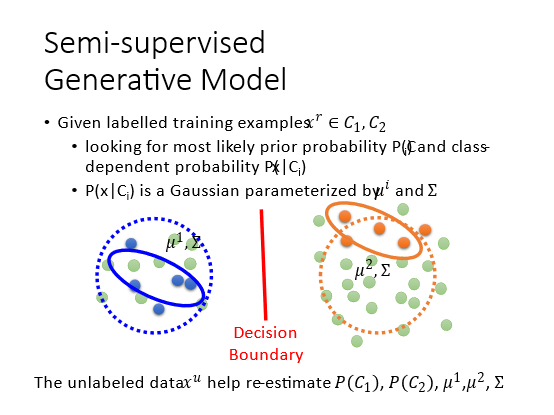1. 初始化一组参数 θ = { P ( C 1 ) , P ( C 2 ) , μ 1 , μ 2 , Σ } 。  \theta=\left\{P\left(C_{1}\right), P\left(C_{2}\right), \mu^{1}, \mu^{2}, \Sigma\right\}_{\text {。 }}
2. 计算每笔无标签数据的后验概率(posterior probability) P θ ( C 1 ∣ x u ) , x u P_{\theta}\left(C_{1} \mid x^{u}\right), x^{u} 表示无标签的数 据。
3. 通过 N 1 + ∑ x u P ( C 1 ∣ x u ) N ( N \frac{N_{1}+\sum_{x^{u}} P\left(C_{1} \mid x^{u}\right)}{N}\left(N\right. 是所有样本的数量, N 1 N_{1} 是被标记为 C 1 C_{1} 的样本数量)来更新 P ( C 1 ) P\left(C_{1}\right) ， 其中 C 1 C_{1} 出现的次数就是所有无标签数据属于 C 1 C_{1} 的概率之和。
μ 1 = 1 N 1 ∑ x r ∈ C 1 x r + 1 ∑ x u P ( C 1 ∣ x u ) ∑ x u P ( C 1 ∣ x u ) x u … … \mu^{1}=\frac{1}{N_{1}} \sum_{x^{r} \in C_{1}} x^{r}+\frac{1}{\sum_{x^{u}} P\left(C_{1} \mid x^{u}\right)} \sum_{x^{u}} P\left(C_{1} \mid x^{u}\right) x^{u} \ldots \ldots
μ 1 \mu^{1} 通过上面的公式更新(等式右边第一个式子是计算所有属于 C 1 C_{1} 的样本的均值, 第二个式子, 如果 x u x^{u} 偏向于 C 1 C_{1} , 那么就对 P ( C 1 ) P\left(C_{1}\right) 的影响就大一点, 反之就小一点。把它们加起来，再除以所 有 x u x_{u} P ( C 1 ∣ x u ) P\left(C_{1} \mid x^{u}\right) 的和)。
4. 有了新的参数后就可以回到第一步(EM算法)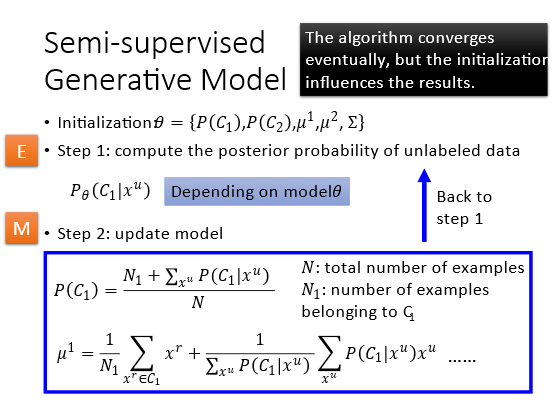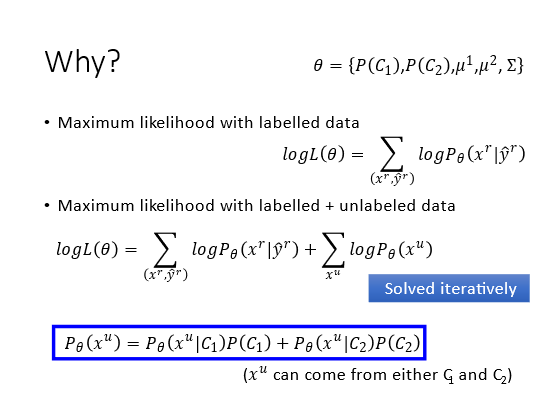1. 假设原来只有有标签数据, 我们要做的事情是最大化似然log L ( θ ) = ∑ x r log ⁡ P θ ( x r , y ^ r ) L(\theta)=\sum_{x^{r}} \log P_{\theta}\left(x^{r}, \hat{y}^{r}\right) , 如
果给定参数 θ \theta , 那么每笔训练数据的 P θ ( x r , y ^ r ) P_{\theta}\left(x^{r}, \hat{y}^{r}\right) 是可以算出来的： P θ ( x r , y ^ r ) = P_{\theta}\left(x^{r}, \hat{y}^{r}\right)=
P θ ( x r ∣ y ^ r ) P ( y ^ r ) P_{\theta}\left(x^{r} \mid \hat{y}^{r}\right) P\left(\hat{y}^{r}\right)
2. 现在同时有有标签数据和无标签数据 使用log L ( θ ) = ∑ x r log ⁡ P θ ( x r , y ^ r ) + L(\theta)=\sum_{x^{r}} \log P_{\theta}\left(x^{r}, \hat{y}^{r}\right)+
∑ x u log ⁡ P θ ( x u ) \sum_{x^{u}} \log P_{\theta}\left(x^{u}\right) , 其中一笔无标签数据出现的几率 P θ ( x u ) = P θ ( x u ∣ C 1 ) P ( C 1 ) + P_{\theta}\left(x^{u}\right)=P_{\theta}\left(x^{u} \mid C_{1}\right) P\left(C_{1}\right)+
P θ ( x u ∣ C 2 ) P ( C 2 ) P_{\theta}\left(x^{u} \mid C_{2}\right) P\left(C_{2}\right) 就是 C 1 C_{1} 的先验概率乘以 C 1 C_{1} 类别产生无标签数据的概率加上 C 2 C_{2} 的先验概率乘 以 C 2 C_{2} 类别产生无标签数据的概率（全概率公式）。就是说这笔无标签数据可能从 C 1 C_{1} 来，也可能 从 C 2 C_{2} 中来, 接下来就要最大化log L ( θ ) = ∑ x r log ⁡ P θ ( x r , y ^ r ) + ∑ x u log ⁡ P θ ( x u ) L(\theta)=\sum_{x^{r}} \log P_{\theta}\left(x^{r}, \hat{y}^{r}\right)+\sum_{x^{u}} \log P_{\theta}\left(x^{u}\right)

# 3 低密度分隔假设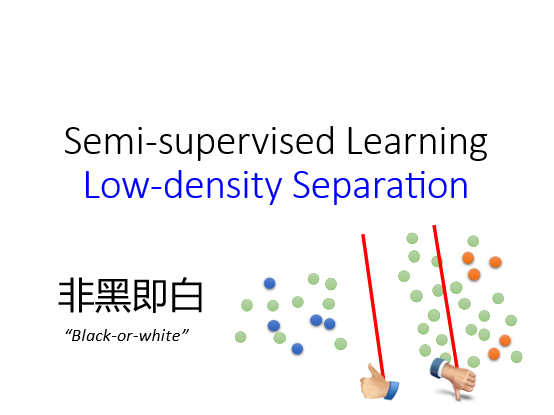## 3.1 自训练算法（Self-training）

1. 先用有标签的数据训练出一个模型。
2. 再把这个模型应用于无标签的数据，看输出的标签是什么，这些标签称为伪标签。
3. 从无标签的数据中拿一些数据去训练模型，再把得到的数据放回标签数据集中。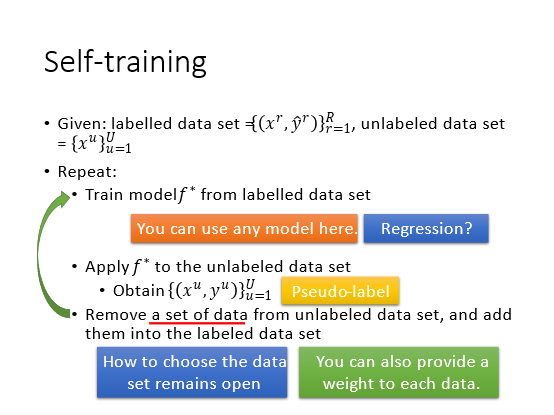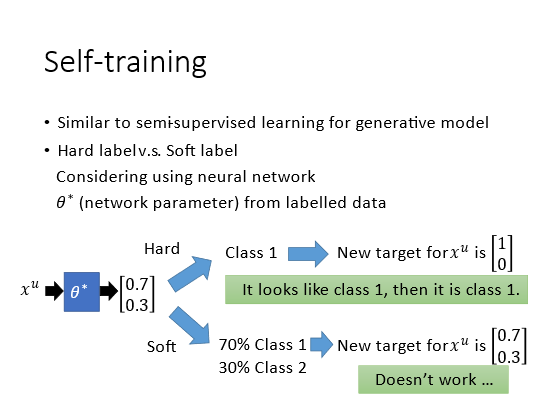## 3.2 基于熵的正则化(Entropy-based Regularization)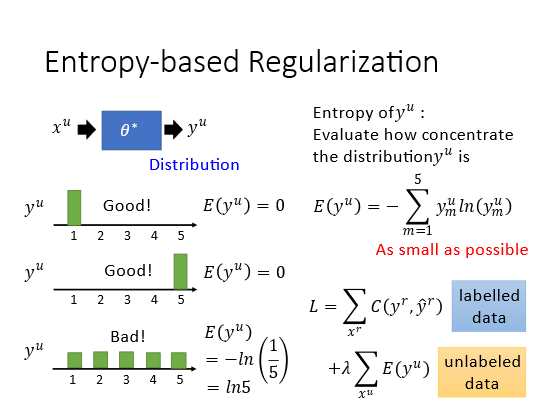E ( y u ) = − ∑ m = 1 N y m u ln ⁡ ( y m u ) E\left(y^{u}\right)=-\sum_{m=1}^{N} y_{m}^{u} \ln \left(y_{m}^{u}\right)

L = ∑ x r C ( y r , y ^ r )  labelled   data  + λ ∑ x u E ( y u )  unlabeled   data  \begin{aligned} L &=\sum_{x^{r}} C\left(y^{r}, \hat{y}^{r}\right) & \begin{array}{c} \text { labelled } \\ \text { data } \end{array} \\ &+\lambda \sum_{x^{u}} E\left(y^{u}\right) & \begin{array}{c} \text { unlabeled } \\ \text { data } \end{array} \end{aligned}

## 3.3 半监督的SVM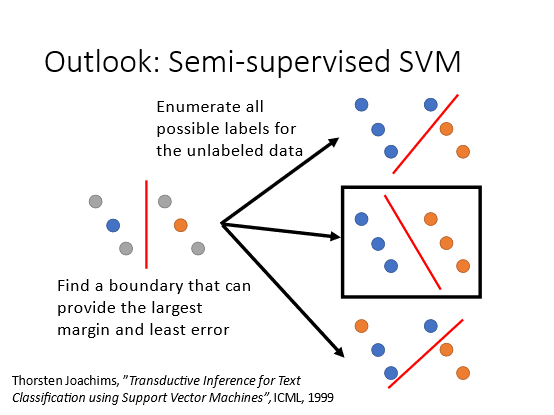# 4 平滑性假设（Smoothness Assumption）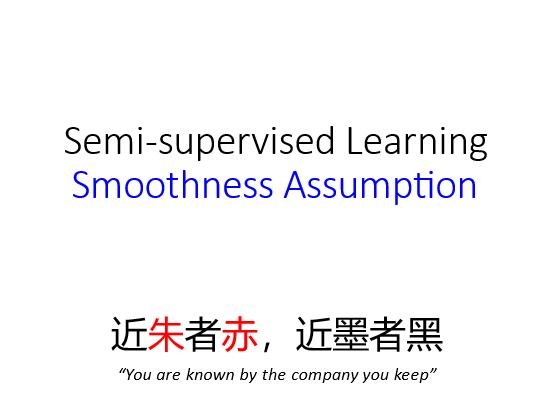• x x 的分布是不平均的
• 如果 x 1 x^{1} x 2 x^{2} 都空间分布中在同一个高密度的区域, 那么就假设有 x 1 x^{1} x 2 x^{2} 有相同的label。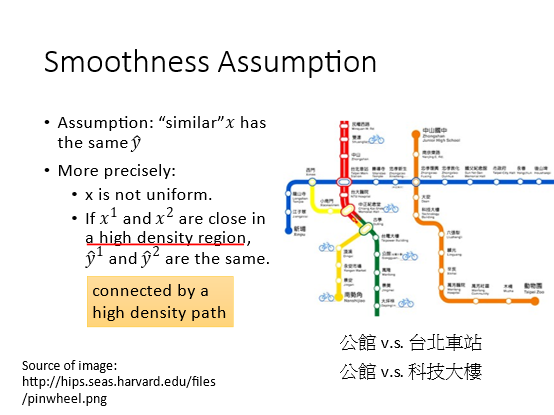## 4.1 基于密度的方式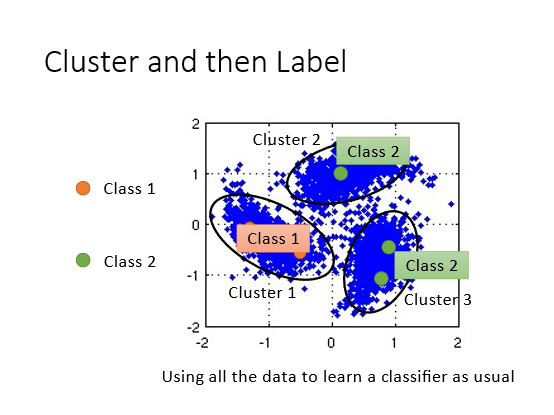## 4.2 基于图的方式(Graph-based Approach)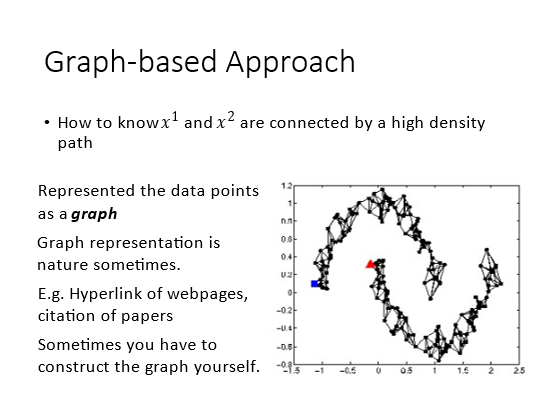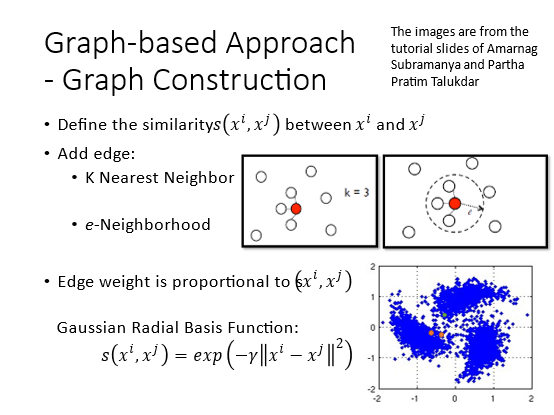有标签的数据会影响他的邻居，类别信息会传递。但是数据要足够多，才能传过去：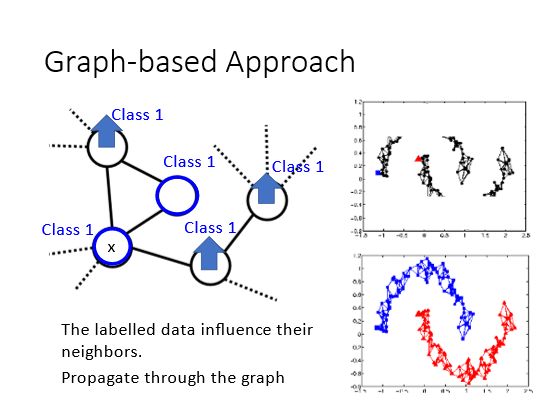Smoothness function的假设

S = 1 2 ∑ i , j w i , j ( y i − y j ) 2 = y T L y S=\frac{1}{2} \sum_{i, j} w_{i, j}\left(y^{i}-y^{j}\right)^{2}=\boldsymbol{y}^{T} L \boldsymbol{y}
S的值越小越平滑：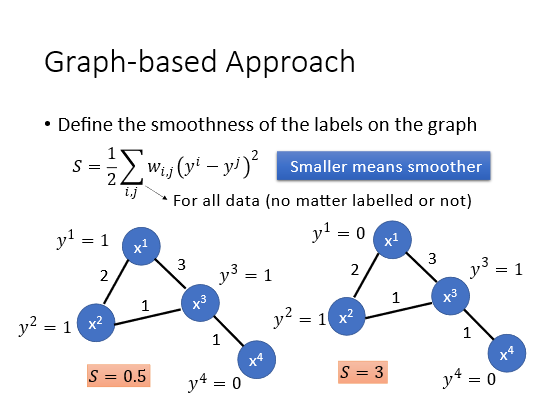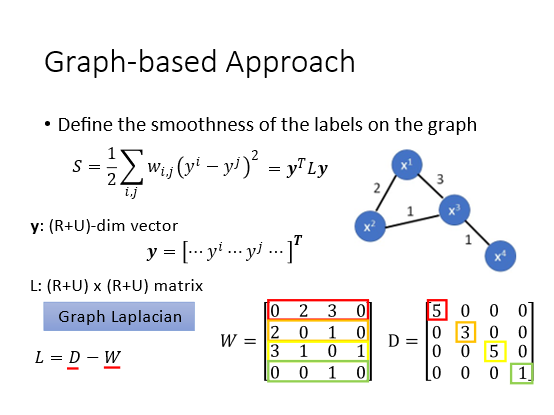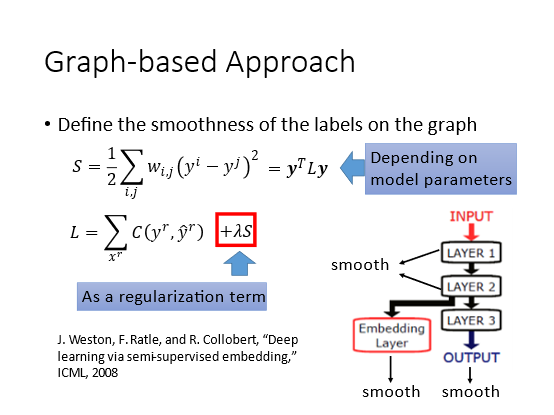可以放在哪一层都可以。

1. 李宏毅老师深度学习视频课2020
3. https://blog.csdn.net/dukuku5038/article/details/82929071

### 热门文章## Java输出数组的内容## 母螳螂的“魅惑之术”## 疯狂填词 mad_libs 第9章9.9.2

#win7 python3.7.0 import os,reos.chdir(d:\documents\program_language) file1open(.\疯狂填词_d9z9d2_r.txt) file2open(.\疯狂填词_d9z9d2_w.txt,w) words[ADJECTIVE,NOUN,VERB,NOUN] str1file1.read()#方法1 for word in words :word_replaceinput(fEnter a {word} :)str1…## 分享你的看书记录

1、软件评测师教程&#xff08;纸质版&#xff09;共19章 2022.01.16 第1-7章、15章看完## HBASE 高可用## js事件操作语法## Photoshop插件--晕影动态--选区--脚本开发--PS插件## SQL练习问题汇总_及时更新## vs LNK1104 无法打开文件“xxx.obj”## 工业元宇宙的定义与实施路线图## 【leectode 2022.1.15】完成一半题目## js 面试题总结## java练习代码## RocketMQ-什么是死信队列？怎么解决## 项目 cg day04## 输出三角形

#include <stdio.h> int main() { int i,j; for(i0;i<5;i) { for(j0;j<i;j) { printf("*"); } printf("\n"); } }## 通过awk和shell来限制IP多次访问之学不会你打死我## Python - 如何像程序员一样思考